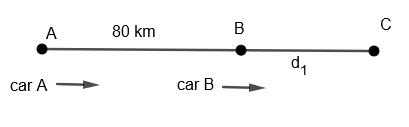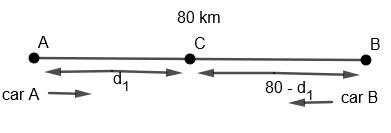Courses
Courses for Kids
Free study material
Offline Centres
MoreLast updated date: 24th Nov 2023
Total views: 381.3k
Views today: 4.81k

# Places A and B are 80 km apart from each other on a highway. A car starts from A and other from B at the same time. If they move in the same direction, they meet in 8 hours and if they move in opposite directions, they meet in 1 hour and 20 minutes. Find the speed of the car.Verified
381.3k+ views
Hint: First of all, consider speed of car A and car B as ${{S}_{A}}$ and ${{S}_{B}}$ respectively. When they are moving in same direction, use $\left( {{S}_{A}}-{{S}_{B}} \right)=\dfrac{\text{Distance between them}}{\text{Time taken by them to meet}}$. Solve these two equations to find ${{S}_{A}}$ and ${{S}_{B}}$.

We are given that place A and B are 80 km apart. Also A and B starts at the same time from positions A and B respectively. When they move in the same direction, they meet in 8 hours and when they move in the opposite direction that is towards each other they meet in 1 hour 20 minutes. We have to find the speeds of car A and B.
Let us diagrammatically consider the situation,
Case 1: When both cars are moving in the same direction.Let us consider that these cars meet at point C.
Let $BC={{d}_{1}}$
Let the speed of car A be ${{S}_{A}}$ and speed of car B be ${{S}_{B}}$.
In this case, we are given that car A and car B meet after 8 hours. Since they meet at C, therefore this means that car A takes 8 hours to reach C and car B also takes 8 hours to reach C. Hence, we get,
Time taken by car A to reach car C = Time taken by car B to reach C = 8 hours.
As we know that time taken $=\dfrac{\text{Distance travelled}}{\text{Speed}}$
Therefore, we get time taken by car A to reach car C $=\dfrac{\text{Distance travelled by car A}}{\text{Speed of car A}}$
By putting the value of distance travelled = AB + BC = $80+{{d}_{1}}$
Speed of car A $={{S}_{A}}$ and time taken = 8 hours, we get
$\dfrac{80+{{d}_{1}}}{{{S}_{A}}}=8.....\left( i \right)$
Also, we get time taken by car B to reach C $=\dfrac{\text{Distance travelled by car B}}{\text{Speed of car B}}$
By putting the value of distance travelled $={{d}_{1}}$
Speed of car $={{S}_{B}}$ and time taken = 8 hours, we get,
$\dfrac{{{d}_{1}}}{{{S}_{B}}}=8$
By cross multiplying above equation, we get
${{d}_{1}}=8{{S}_{B}}$
By putting the value of ${{d}_{1}}$ in equation (i), we get,
$\dfrac{80+8{{S}_{B}}}{{{S}_{A}}}=8$
By cross multiplying the above equation, we get,
$80+8{{S}_{B}}=8{{S}_{A}}$
$8{{S}_{A}}-8{{S}_{B}}=80$
By taking out 8 common in above equation, we get,
${{S}_{A}}-{{S}_{B}}=10....\left( ii \right)$

Case 2: When cars are moving towards each other or in opposite directionLet us consider that these cars meet at C.
Let $AC={{d}_{1}}$
Since, AC + CB = 80 km
Therefore, $CB=80-AC=\left( 80-{{d}_{1}} \right)km$
In this case, we are given that car A and car B meet after 1 hour 20 minutes $=\left( 1+\dfrac{20}{60} \right)\text{hours}=1+\dfrac{1}{3}=\dfrac{4}{3}\text{hours}$
Since, they meet at C, therefore this means that car A takes $\dfrac{4}{3}$ hours to reach C and car B also takes $\dfrac{4}{3}$ hours to reach C. Hence, we get
Time taken by car A to reach C = Time taken by car B to reach C = $\dfrac{4}{3}$ hours.
As, we know that time taken $=\dfrac{\text{distance travelled}}{\text{speed}}$
Therefore, we get time taken by car A to reach car C $=\dfrac{\text{Distance travelled by car A}}{\text{Speed of car A}}$
By putting the value of distance travelled $=AC={{d}_{1}}$
Speed of car A $={{S}_{A}}$ and time taken $=\dfrac{4}{3}\text{hours}$, we get
$\dfrac{{{d}_{1}}}{{{S}_{A}}}=\dfrac{4}{3}$
By cross multiplying above equation, we get
${{d}_{1}}=\dfrac{4{{S}_{A}}}{3}....\left( iii \right)$
Also, we get the time taken by car B to reach C $=\dfrac{\text{Distance travelled by car B}}{\text{Speed of car B}}$
By putting the value of distance travelled $=BC=80-{{d}_{1}}$
Speed of car A $={{S}_{B}}$ and time taken $=\dfrac{4}{3}\text{hours}$, we get
$\dfrac{80-{{d}_{1}}}{{{S}_{B}}}=\dfrac{4}{3}$
By cross multiplying above equation, we get,
$3\left( 80-{{d}_{1}} \right)=4{{S}_{B}}$
By putting the value of ${{d}_{1}}$ from equation (iii), we get
$3\left( 80-\dfrac{4{{S}_{A}}}{3} \right)=4{{S}_{B}}$
$=3\times 80-4{{S}_{A}}-4{{S}_{B}}$
Or, $4\left( {{S}_{A}}+{{S}_{B}} \right)=240$
By dividing by 4 on both sides, we get
$\left( {{S}_{A}}+{{S}_{B}} \right)=60....\left( iv \right)$
By adding equation (ii) and (iv), we get
$\left( {{S}_{A}}+{{S}_{B}} \right)+\left( {{S}_{A}}-{{S}_{B}} \right)=60+10$
$\Rightarrow 2{{S}_{A}}=70$
Therefore, we get ${{S}_{A}}=\dfrac{70}{2}=35km/hr$
By putting the value of ${{S}_{A}}$ in equation (iv), we get
\begin{align} & 35+{{S}_{B}}=60 \\ & \Rightarrow {{S}_{B}}=60-35=25km/hr \\ \end{align}
Therefore, we get the speed of car A as 35 km/hr and car B as 25 km/hr.

Note: Students must note that whenever two cars are moving in the same direction, they will never meet at a point between them. In the same way, if they are moving towards each other, they will always meet a point between them. Also, students can cross check their answer by putting ${{S}_{A}}$ and ${{S}_{B}}$ back into the equation and checking if they are satisfying the equations or not.
Here, students can also calculate ${{S}_{A}}$ and ${{S}_{B}}$ by using direct formula, that is ${{S}_{A}}=\dfrac{\dfrac{D}{{{T}_{1}}}+\dfrac{D}{{{T}_{2}}}}{2}$ and ${{S}_{B}}=\dfrac{\dfrac{D}{{{T}_{1}}}+\dfrac{D}{{{T}_{2}}}}{2}$ where D is the distance between A and B, ${{T}_{1}}$ is the time taken by them to meet when they are moving towards each other and ${{T}_{2}}$ is the time taken by them to meet when they are moving in the same direction.